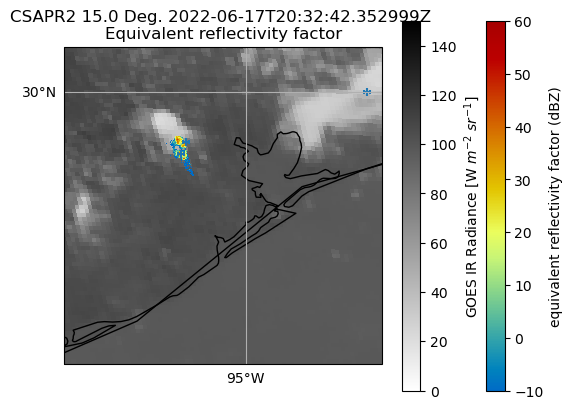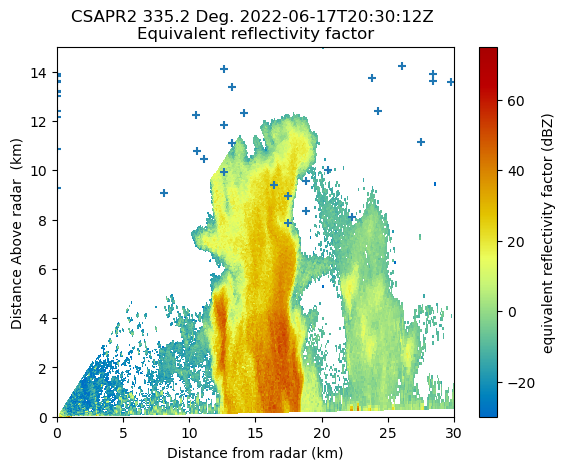# Blending Observations from TRACER using Py-ART#

The TRacking Aerosol Convection Interactions (TRACER) field experiment was a U.S. Department of Energy IOP with the goal of studying the lifecycle of convection over Houston as well as potential aerosol impacts on this lifecycle. Houston is uniquely suited for this kind of field experiment where seabreeze convection forms off of the coast of Houston in cleaner air conditions and then approaches the more polluted Houston region. For more information about the TRACER field experiment, click here.

This post will show how to plot overlays of Texas A&M University Lightning Mapping Array data over GOES and ARM CSAPR2 data for a case of wildfire smoke entraining into developing convection sampled during July 12 and 13, 2022. In addition, we highlight a case that was tracked by CSAPR2 for 90 minutes on June 17, 2022.

## Imports#

import pyart
import act
import matplotlib.pyplot as plt
import xarray as xr
import numpy as np
import s3fs
import cartopy.crs as ccrs
import pandas as pd

from scipy.spatial import KDTree

%matplotlib inline


# GOES data access#

All of the NOAA GOES satellite data are available on Amazon Web Services for download. Therefore, you can use the s3fs tool to download the required data. We will download band 13, which is the longwave infrared band of GOES. In order to only download the band 13 files, we will ensure that there is a “C13” in the filename as this denotes the band number for each file.

# Use the anonymous credentials to access public data
fs = s3fs.S3FileSystem(anon=True)

day_no = 168  # Day number of year (here is June 17th, 16th on leap year)
year = 2022
hour = 20
files = np.array(fs.ls("noaa-goes16/ABI-L1b-RadC/%d/%d/%02d/" % (year, day_no, hour)))
band = "13"
for fi in files:
if "C13" in fi:
fs.get(fi, "../data/" + fi.split("/")[-1])

Downloading noaa-goes16/ABI-L1b-RadC/2022/168/20/OR_ABI-L1b-RadC-M6C13_G16_s20221682001178_e20221682003562_c20221682004026.nc

goes_ds = xr.open_dataset(
)
goes_ds

<xarray.Dataset>
Dimensions:                                           (y: 1500, x: 2500,
number_of_time_bounds: 2,
number_of_image_bounds: 2,
band: 1,
num_star_looks: 24)
Coordinates:
t                                                 datetime64[ns] 2022-07-...
* y                                                 (y) float64 0.1282 ... ...
* x                                                 (x) float64 -0.1013 ......
y_image                                           float32 0.08624
x_image                                           float32 -0.03136
band_id                                           (band) int8 13
band_wavelength                                   (band) float32 10.33
t_star_look                                       (num_star_looks) datetime64[ns] ...
band_wavelength_star_look                         (num_star_looks) float32 ...
Dimensions without coordinates: number_of_time_bounds, number_of_image_bounds,
band, num_star_looks
Data variables: (12/37)
DQF                                               (y, x) float32 ...
time_bounds                                       (number_of_time_bounds) datetime64[ns] ...
goes_imager_projection                            int32 -2147483647
y_image_bounds                                    (number_of_image_bounds) float32 ...
x_image_bounds                                    (number_of_image_bounds) float32 ...
...                                                ...
algorithm_dynamic_input_data_container            int32 -2147483647
processing_parm_version_container                 int32 -2147483647
algorithm_product_version_container               int32 -2147483647
star_id                                           (num_star_looks) float32 ...
channel_integration_time                          float64 336.0
channel_gain_field                                float64 0.0
Attributes: (12/30)
naming_authority:          gov.nesdis.noaa
Conventions:               CF-1.7
standard_name_vocabulary:  CF Standard Name Table (v35, 20 July 2016)
institution:               DOC/NOAA/NESDIS > U.S. Department of Commerce,...
project:                   GOES
production_site:           WCDAS
...                        ...
timeline_id:               ABI Mode 6
date_created:              2022-07-12T20:09:02.3Z
time_coverage_start:       2022-07-12T20:06:17.3Z
time_coverage_end:         2022-07-12T20:08:55.7Z
LUT_Filenames:             SpaceLookParams(FM1A_CDRL79RevP_PR_09_00_02)-6...
id:                        f45b564c-dcb9-498a-ac56-da80a1d735a5

The following code in Python was created by the NOAA/NESDIS/STAR Aerosols and Atmospheric Composition Science Team to convert the GOES projection values to lat/lon coordinates that are more useful for selecting a sub-domain of GOES to plot. The original code used the netCDF4 library, so it was adapted to xarray by Bobby Jackson.

# Calculate latitude and longitude from GOES ABI fixed grid projection data
# GOES ABI fixed grid projection is a map projection relative to the GOES satellite
# Units: latitude in °N (°S < 0), longitude in °E (°W < 0)
# See GOES-R Product User Guide (PUG) Volume 5 (L2 products) Section 4.2.8 for details & example of calculations
# "file_id" is an ABI L1b or L2 .nc file opened using the netCDF4 library

def calculate_degrees(file_id):

# Read in GOES ABI fixed grid projection variables and constants
x_coordinate_1d = file_id.variables["x"][:]  # E/W scanning angle in radians
y_coordinate_1d = file_id.variables["y"][:]  # N/S elevation angle in radians
projection_info = file_id.variables["goes_imager_projection"]
lon_origin = projection_info.attrs["longitude_of_projection_origin"]
H = (
projection_info.attrs["perspective_point_height"]
+ projection_info.attrs["semi_major_axis"]
)
r_eq = projection_info.attrs["semi_major_axis"]
r_pol = projection_info.attrs["semi_minor_axis"]

# Create 2D coordinate matrices from 1D coordinate vectors
x_coordinate_2d, y_coordinate_2d = np.meshgrid(x_coordinate_1d, y_coordinate_1d)

# Equations to calculate latitude and longitude
lambda_0 = (lon_origin * np.pi) / 180.0
a_var = np.power(np.sin(x_coordinate_2d), 2.0) + (
np.power(np.cos(x_coordinate_2d), 2.0)
* (
np.power(np.cos(y_coordinate_2d), 2.0)
+ (
((r_eq * r_eq) / (r_pol * r_pol))
* np.power(np.sin(y_coordinate_2d), 2.0)
)
)
)
b_var = -2.0 * H * np.cos(x_coordinate_2d) * np.cos(y_coordinate_2d)
c_var = (H**2.0) - (r_eq**2.0)
r_s = (-1.0 * b_var - np.sqrt((b_var**2) - (4.0 * a_var * c_var))) / (2.0 * a_var)
s_x = r_s * np.cos(x_coordinate_2d) * np.cos(y_coordinate_2d)
s_y = -r_s * np.sin(x_coordinate_2d)
s_z = r_s * np.cos(x_coordinate_2d) * np.sin(y_coordinate_2d)

# Ignore numpy errors for sqrt of negative number; occurs for GOES-16 ABI CONUS sector data
np.seterr(all="ignore")

abi_lat = (180.0 / np.pi) * (
np.arctan(
((r_eq * r_eq) / (r_pol * r_pol))
* ((s_z / np.sqrt(((H - s_x) * (H - s_x)) + (s_y * s_y))))
)
)
abi_lon = (lambda_0 - np.arctan(s_y / (H - s_x))) * (180.0 / np.pi)

return abi_lat, abi_lon


Calculate the latitude and longitude coordinates of the GOES data and then crop the data to our region of interest: 25 to 35 $$^{\circ} N$$ and 100 to 95 $$^{\circ} W$$.

lat, lon = calculate_degrees(goes_ds)
lat = np.nan_to_num(lat, -9999)
lon = np.nan_to_num(lon, -9999)
lat_range = (25.0, 35.0)
lon_range = (-100.0, -95.0)
lat_min = lat.min(axis=1)
lat_max = lat.max(axis=1)
lat_min_bound = np.argmin(np.abs(lat_min - lat_range))
lat_max_bound = np.argmin(np.abs(lat_max - lat_range))
lon_min = lon.min(axis=0)
lon_max = lon.max(axis=0)
lon_min_bound = np.argmin(np.abs(lon_min - lon_range))
lon_max_bound = np.argmin(np.abs(lon_max - lon_range))


# CSAPR data access#

The a1-level CSAPR data are available for public use on ARM Data Disovery. In addition, we can use the Atmospheric Community Toolkit to download the July 12 and 13 CSAPR data. The DOI for the CSAPR2 a1-level data is 10.5439/1467901, which if accessed will bring you to the download page on ARM Data Discovery.

The first block of code below will look at the automated catalogue of CSAPR data created by Adam Theisen. This provides us with a view of what scanning mode the CSAPR was in for a given time period as well as the scanning geometry and precipitation coverage.

cell_track_info = pd.read_csv(
index_col=["time"],
parse_dates=True,
)
cell_track_info

Unnamed: 0 scan_mode scan_name template_name azimuth_min azimuth_max elevation_min elevation_max range_min range_max cell_azimuth cell_range cell_zh ngates_gt_0 ngates_gt_10 ngates_gt_30 ngates_gt_50 ngates_gt_10_5km ngates_gt40_5km ngates
time
2022-06-17 00:00:03 0 rhi rhi hou-rhi-cell-track-05-deg 303.74450 303.74450 0.637207 4.383545 0.0 109900.0 NaN NaN NaN 0 0 0 0 0 0 0
2022-06-17 00:06:09 1 ppi ppi hou-ppi-cell-track-05-deg 297.59216 307.63367 3.021240 3.988037 0.0 109900.0 297.910767 700.0 30.930866 59 30 3 0 0 0 78
2022-06-17 00:06:40 2 rhi rhi hou-rhi-cell-track-05-deg 302.64587 302.64587 0.637207 4.383545 0.0 109900.0 302.645874 1800.0 -0.776333 0 0 0 0 0 0 2
2022-06-17 00:06:59 3 rhi rhi hou-rhi-cell-track-05-deg 302.55798 302.59094 0.637207 4.383545 0.0 109900.0 NaN NaN NaN 0 0 0 0 0 0 0
2022-06-17 00:07:18 4 rhi rhi hou-rhi-cell-track-05-deg 302.97546 302.99744 0.637207 4.383545 0.0 109900.0 302.975464 900.0 -2.972101 0 0 0 0 0 0 2
... ... ... ... ... ... ... ... ... ... ... ... ... ... ... ... ... ... ... ... ...
2022-06-17 23:39:46 2826 rhi rhi hou-rhi-cell-track-10-deg 301.48132 301.50330 0.648193 9.382324 0.0 109900.0 301.503296 62400.0 40.327583 990 989 210 0 134 0 995
2022-06-17 23:40:02 2827 rhi rhi hou-rhi-cell-track-10-deg 305.70007 305.70007 0.648193 9.404297 0.0 109900.0 305.700073 13600.0 29.037998 12 6 0 0 0 0 20
2022-06-17 23:40:16 2828 rhi rhi hou-rhi-cell-track-10-deg 297.23510 297.26807 0.648193 9.382324 0.0 109900.0 297.235107 700.0 28.459156 28 25 0 0 0 0 34
2022-06-17 23:40:29 2829 ppi ppi hou-ppi-cell-track-10-deg 296.08704 306.16150 2.999268 6.998291 0.0 109900.0 300.646362 59100.0 33.882927 807 783 27 0 63 0 830
2022-06-17 23:40:54 2830 rhi rhi hou-rhi-cell-track-10-deg 301.05835 301.08032 0.648193 9.382324 0.0 109900.0 301.058350 62800.0 37.198204 767 767 75 0 76 0 773

2831 rows × 20 columns

For this notebook we are interested in the time periods where the CSAPR was scanning in PPI mode. For this entire time period, the CSAPR was in cell-tracking mode, meaning that it was focused on a single convective cell in the Houston region. This scanning strategy will have a much more frequent scans over a smaller area covered by a single convective cell and is designed to track the lifecycle of a convective cell.

start_hour = 20
end_hour = 21
print("All PPI scan times between %d UTC and %d UTC:" % (start_hour, end_hour))
for index in cell_track_info.index:
if index.hour >= 20 and index.hour < end_hour:
if cell_track_info.loc[index].scan_mode == "ppi":
print(index)

All PPI scan times between 20 UTC and 21 UTC:
2022-06-17 20:00:07
2022-06-17 20:00:51
2022-06-17 20:01:34
2022-06-17 20:02:17
2022-06-17 20:03:05
2022-06-17 20:03:48
2022-06-17 20:04:31
2022-06-17 20:05:22
2022-06-17 20:06:08
2022-06-17 20:06:59
2022-06-17 20:07:43
2022-06-17 20:08:29
2022-06-17 20:09:15
2022-06-17 20:09:56
2022-06-17 20:10:53
2022-06-17 20:11:39
2022-06-17 20:14:24
2022-06-17 20:16:25
2022-06-17 20:18:27
2022-06-17 20:20:25
2022-06-17 20:22:31
2022-06-17 20:24:30
2022-06-17 20:26:31
2022-06-17 20:28:30
2022-06-17 20:30:31
2022-06-17 20:32:29
2022-06-17 20:34:30
2022-06-17 20:36:30
2022-06-17 20:38:31
2022-06-17 20:40:32
2022-06-17 20:42:33
2022-06-17 20:44:31
2022-06-17 20:46:31
2022-06-17 20:48:25
2022-06-17 20:50:27
2022-06-17 20:52:21
2022-06-17 20:54:20
2022-06-17 20:56:22
2022-06-17 20:57:51
2022-06-17 20:59:19

csapr = pyart.io.read(
)


Here we load the data from the Houston Lightning Mapping Array. The data are in standard netCDF format that is easily read by xarray. The data are currently available for order at https://data.eol.ucar.edu/cgi-bin/codiac/fgr_form/id=100.016. Both the flash density data and locations of each flash are stored in the file.

lma_ds = xr.open_dataset(
)


## June 17th case#

This is a case of a storm that was tracked by CSAPR for about 90 minutes during the afternoon of June 17th 2022. We will use the same code from above to plot the radar/LMA overlays over the GOES IR radiance data for this case. This first block of code combines the above code to load and subset all of the necessary data for the overlays.

goes_ds = xr.open_dataset(
)
)
lma_ds = xr.open_dataset(
)
lma_ds
lat, lon = calculate_degrees(goes_ds)
lat = np.nan_to_num(lat, -9999)
lon = np.nan_to_num(lon, -9999)
lat_range = (25.0, 35.0)
lon_range = (-100.0, -95.0)
lat_min = lat.min(axis=1)
lat_max = lat.max(axis=1)
lat_min_bound = np.argmin(np.abs(lat_min - lat_range))
lat_max_bound = np.argmin(np.abs(lat_max - lat_range))
lon_min = lon.min(axis=0)
lon_max = lon.max(axis=0)
lon_min_bound = np.argmin(np.abs(lon_min - lon_range))
lon_max_bound = np.argmin(np.abs(lon_max - lon_range))


Here, we use cartopy to plot the GOES/LMA data and then the RadarMapDisplay object to overlay the radar data on top of the GOES/LMA data.

fig, ax = plt.subplots(1, 1, subplot_kw=dict(projection=ccrs.PlateCarree()))
gatefilter = pyart.filters.GateFilter(csapr)
gatefilter.exclude_below("normalized_coherent_power", 0.50)

c = ax.pcolormesh(
lon[lat_max_bound:lat_min_bound, lon_max_bound:lon_min_bound],
lat[lat_max_bound:lat_min_bound, lon_max_bound:lon_min_bound],
cmap="binary",
vmin=0,
vmax=150,
)
disp.plot_ppi_map(
"reflectivity", gatefilter=gatefilter, ax=ax, sweep=1, vmin=-10, vmax=60
)

plt.colorbar(c, label="GOES IR Radiance [W $m^{-2}\ sr^{-1}]$")

flash_times = np.squeeze(
np.argwhere(
np.logical_and(
lma_ds.flash_time_start.values > np.datetime64("2022-06-17T20:31:00"),
lma_ds.flash_time_start.values < np.datetime64("2022-06-17T20:33:00"),
)
)
)

ax.scatter(
lma_ds.flash_center_longitude[flash_times],
lma_ds.flash_center_latitude[flash_times],
marker="+",
)
ax.coastlines()
ax.set_xlim([-96, -94.25])
ax.set_ylim([28.5, 30.25])

<>:14: DeprecationWarning: invalid escape sequence '\ '
<>:14: DeprecationWarning: invalid escape sequence '\ '
/var/folders/xf/43jvg_v90fx7z1sj2j1v8h0w0000gn/T/ipykernel_41252/2371313213.py:14: DeprecationWarning: invalid escape sequence '\ '
plt.colorbar(c, label='GOES IR Radiance [W $m^{-2}\ sr^{-1}]$')
/Users/rjackson/opt/anaconda3/envs/pyart_env2/lib/python3.10/site-packages/cartopy/mpl/gridliner.py:451: UserWarning: The .xlabels_top attribute is deprecated. Please use .top_labels to toggle visibility instead.
warnings.warn('The .xlabels_top attribute is deprecated. Please '
/Users/rjackson/opt/anaconda3/envs/pyart_env2/lib/python3.10/site-packages/cartopy/mpl/gridliner.py:487: UserWarning: The .ylabels_right attribute is deprecated. Please use .right_labels to toggle visibility instead.
warnings.warn('The .ylabels_right attribute is deprecated. Please '

(28.5, 30.25)## June 17 RHI#

We are also interested in the Range-Height Indicator scans provided by CSAPR2 as this cell evolves. The RHI scans will provide a vertical-cross section of the microphysical properties of the storm as it evolves. Finally, this block of code will plot the altitudes and locations of each lightning strike overlaid over the RHI scan. SciPy’s KDTree is used to find the nearest neighbors between the LMA lightning strike locations and the latitude and longitude locations of each gate in the RHI. After the nearest neighbors are found this provides us the altitude and range where the approximate location of the lightning strike would be.

csapr_rhi = pyart.io.read(
)
gatefilter = pyart.filters.GateFilter(csapr_rhi)
gatefilter.exclude_below("normalized_coherent_power", 0.50)

flash_times = np.squeeze(
np.argwhere(
np.logical_and(
lma_ds.flash_time_start.values > np.datetime64("2022-06-17T20:30:00"),
lma_ds.flash_time_start.values < np.datetime64("2022-06-17T20:31:00"),
)
)
)
flash_alts = lma_ds.flash_init_altitude[flash_times]
flash_lons = lma_ds.flash_init_longitude[flash_times]
flash_lats = lma_ds.flash_init_latitude[flash_times]
rhi_lons = csapr_rhi.gate_longitude["data"].flatten()
rhi_lats = csapr_rhi.gate_latitude["data"].flatten()
rhi_alts = csapr_rhi.gate_altitude["data"].flatten()

kdtree_data = np.stack([rhi_lons, rhi_lats], axis=1)
kdtree = KDTree(kdtree_data)
inp_data = np.stack([flash_lons, flash_lats], axis=1)
dist, where_in_rhi = kdtree.query(inp_data)

flash_ranges = csapr_rhi.range["data"][where_in_rhi % csapr_rhi.ngates]

(51,)

(0.0, 15.0)